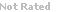Loading... Please wait...

## Top Sellers

• 1
Bulk Blue Struts
\$20.00• 2
Bulk Red Struts
\$20.00• 3
Colored Balls (packs of 30 / 100)
\$40.00• 4
Bulk Yellow Struts
\$20.00• 5
White Balls in Bulk (packs of 30 or 100)
\$16.00# Classifying Triangles —Lesson Module

Classifying Triangles by Line and Angle Properties

Triangles abound in this module! In this series of investigations, students will construct and use a plethora of Zometool triangle models to develop a system of classifying triangles by:
(a) examining the relationships between the side lengths of triangles, or line properties;
(b) examining the relationships between the angle measurements of triangles, or angle properties; and
(c) examining the relationships between the line and angle properties of triangles.

Throughout this module, students will learn to reason with geometric properties in an effort to better identify and understand the major types of triangles that exist in structures around us.

 Primary Content Knowledge/Ideas Focal Practices Triangles can be classified by relationships between line lengths, or line properties: Scalene, Isosceles, and Equilateral Triangles can be classified by relationships between angle measurements, or angle properties: Acute, Right, and Obtuse • Triangles can be identified and classified by relationships between line and angle properties in a triangle: 1. a right isosceles triangle2. a right scalene triangle 3. an acute scalene triangle 4. an acute isosceles triangle 5. an acute equilateral triangle 6. an obtuse scalene triangle 7. an obtuse isosceles triangle Students will identify and reason with relationships between lines and angle properties in triangles to develop a system of classifying triangles. Students will use a variety of tools to visualize, construct, and explain the development of geometric relationships in triangles; ranging from rulers to protractors to Zometool models.

 Grade Levels 3-5 Recommended ages 8-11 SKU ML-LES-TRI File Size: 19.5 MB Pages: 23
Price: \$14.99
Quantity:

• Specs
• Customer Reviews
• Related Products

Classifying Triangles by Line and Angle Properties

Triangles abound in this module! In this series of investigations, students will construct and use a plethora of Zometool triangle models to develop a system of classifying triangles by:
(a) examining the relationships between the side lengths of triangles, or line properties;
(b) examining the relationships between the angle measurements of triangles, or angle properties; and
(c) examining the relationships between the line and angle properties of triangles.

Throughout this module, students will learn to reason with geometric properties in an effort to better identify and understand the major types of triangles that exist in structures around us.

 Primary Content Knowledge/Ideas Focal Practices Triangles can be classified by relationships between line lengths, or line properties: Scalene, Isosceles, and Equilateral Triangles can be classified by relationships between angle measurements, or angle properties: Acute, Right, and Obtuse • Triangles can be identified and classified by relationships between line and angle properties in a triangle: 1. a right isosceles triangle2. a right scalene triangle 3. an acute scalene triangle 4. an acute isosceles triangle 5. an acute equilateral triangle 6. an obtuse scalene triangle 7. an obtuse isosceles triangle Students will identify and reason with relationships between lines and angle properties in triangles to develop a system of classifying triangles. Students will use a variety of tools to visualize, construct, and explain the development of geometric relationships in triangles; ranging from rulers to protractors to Zometool models.

 Grade Levels 3-5 Recommended ages 8-11 SKU ML-LES-TRI File Size: 19.5 MB Pages: 23

## Related Products

• Geometry of Bubbles —Lesson Module \$14.99## Information